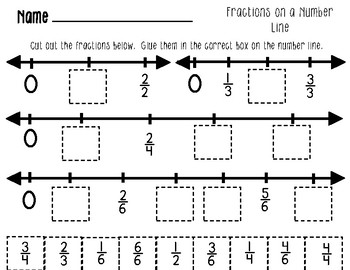Beginning Fractions on a Number LineSubject
Resource Type
Common Core Standards
Product Rating
File Type

PDF (Acrobat) Document File

1 MB|9 pages
Share
Product Description

Keep your students engaged with these hands-on FRACTIONS ON A NUMBER LINE worksheets that can be used for review, homework, guided math, assessing, whole class, or for students needing extra support.

Common Core Standard 3.NF.A.2.

The following worksheets are included:

*Cut and Paste the fractions onto the number line

*Label the number line

*Write the fraction shown on the number line

*Identify the fraction on the number line and paste the matching shaded fraction next to it.

*Color the fraction shown on the number line and label the fractions.

* Create a fraction number line representing the fraction given

* Fraction Match Up - (Cut and Paste/Template) Students match the fraction number, the shaded fraction and the fraction number line.

Other fraction products in my store:

Beginning Fraction Fun Printables

Fraction Flip Book-Introduction to Basic Fractions

Fractions I have - Who Has Loop Game

Beginning Fraction on a Number Line

Total Pages
9 pages